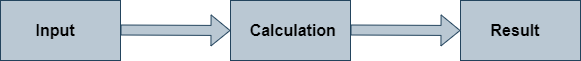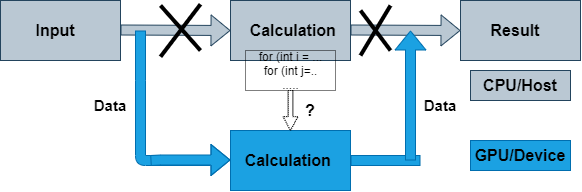# Introduction to OpenACC¶

• Serial Computing on CPU• Porting to GPU## What is OpenACC ?¶

• OpenACC defines a set of compiler directives that allow code regions to be offloaded from a host CPU to be computed on a GPU

• High level GPU programming

• Large similarity to OpenMP directives

• Support for both C/C++ and Fortran bindings

• Extensive guides, tutorials, code samples and documentation on the OpenACC standard can be found at www.openacc.org.

## OpenACC vs. CUDA or HIP¶

• Why OpenACC and not CUDA / HIP?

• Easier to work with

• Porting of existing software requires less work

• Same code can be compiled to CPU and GPU versions easily

• Why CUDA/HIP and not OpenACC?

• More optimization possibilities

## How to port code to GPU with OpenACC?¶

• Compilers that support OpenACC usually require an option that enables the feature

• PGI (now NVIDIA HPC SDK): `-acc`

• Cray: `-hacc`

• GNU (partial support): `-fopenacc`

• Without these options a regular CPU version is compiled!

• OpenACC data model

• host manages memory of the device

• host copies data to/from the device

• OpenACC execution model

• Host-directed execution with an attached accelerator

• Part of the program is usually executed by the host

• Computationally intensive parts are offloaded to the accelerator that executes parallel regions

## OpenACC directive syntax¶

sentinel

construct

clauses

C/C++

`#pragma acc`

`data`

`copy(data)`

data model

data model

execution model

execution model

`update`

`host(data)`

`kernels`

`parallel`

Fortran

`!\$acc`

`data`

`copy(data)`

data model

data model

execution model

execution model

`update`

`host(data)`

`kernels`

`parallel`

• OpenACC uses compiler directives for defining compute regions (and data transfers) that are to be performed on a GPU

• Important constructs

• `parallel`, `kernels`, `data`, `loop`, `update`, `host_data`, `wait`

• Often used clauses

• `if (condition)`, `async(handle)`

## OpenACC data model¶

• Define a region with data declared in the device memory

• C/C++: `#pragma acc data [clauses]`

• Fortran: `!\$acc data [clauses]`

• clauses can be `copy`, `copyin`, `copyout`, and `present`

• Data transfers take place

• from the host to the device upon entry to the region

• from the device to the host upon exit from the region

• Functionality defined by data clauses

• Data clauses can also be used in `kernels` and `parallel` constructs

## OpenACC execution model¶

• OpenACC includes two different approaches for defining parallel regions

• `parallel` defines a region to be executed on an accelerator. Work sharing parallelism has to be defined manually. Good tuning prospects.

• `kernels` defines a region to be transferred into a series of kernels to be executed in sequence on an accelerator. Work sharing parallelism is defined automatically for the separate kernels, but tuning prospects limited.

• With similar work sharing, both can perform equally well

### Compute constructs: `kernels`¶

• Define a region to be transferred to a sequence of kernels for execution on the accelerator device

• C/C++: `#pragma acc kernels [clauses]`

• Fortran: `!\$acc kernels [clauses]`

• Each separate loop nest inside the region will be converted into a separate parallel kernel

• The kernels will be executed in a sequential order

### Compute constructs: `parallel`¶

• Define a region to be executed on the accelerator device

• C/C++: `#pragma acc parallel [clauses]`

• Fortran: `!\$acc parallel [clauses]`

• Without any work sharing constructs, the whole region is executed redundantly multiple times

• Given a sequence of loop nests, each loop nest may be executed

simultaneously

### Work sharing construct: `loop`¶

• Define a loop to be parallelized
• C/C++: `#pragma acc loop [clauses]`

• Fortran: `!\$acc loop [clauses]`

• Must be followed by a C/C++ or Fortran loop construct.

• Combined constructs with `parallel` and `kernels`
• `#pragma acc kernels loop / !\$acc kernels loop`

• `#pragma acc parallel loop / !\$acc parallel loop`

• Similar in functionality to OpenMP `for/do` construct

• Loop index variables are `private` variables by default

## Compiler diagnostics¶

• Compiler diagnostics is usually the first thing to check when starting the OpenACC work

• It can tell you what operations were actually performed

• Data copies that were made

• If and how the loops were parallelized

• The diagnostics is very compiler dependent

• Compiler flags

• Level and formatting of information

• Diagnostics is controlled by compiler flag `-Minfo=option`

• Useful options:

• `accel` – operations related to the accelerator

• `all` – print all compiler output

• `intensity` – print loop computational intensity info

### Example: `-Minfo`¶

```\$ pgcc -g -O3 -acc -Minfo=acc sum_parallel.c -o sum

main:
21, Generating copy(vecA[:],vecB[:],vecC[:]) [if not already present]
23, Generating Tesla code
25, #pragma acc loop gang, vector(128) /* blockIdx.x threadIdx.x */

\$ pgcc -g -O3 -acc -Minfo=accel sum_kernels.c -o sum

main:
21, Generating copy(vecA[:],vecB[:],vecC[:]) [if not already present]
23, Loop is parallelizable
Generating Tesla code
23, #pragma acc loop gang, vector(128) /* blockIdx.x threadIdx.x */
```

### Example: `PGI_ACC_TIME=1`¶

``` \$ cat slurm-13186502.out  #output of sum_parallel.c

Accelerator Kernel Timing data
main  NVIDIA  devicenum=0
time(us): 451
21: data region reached 2 times
21: data copyin transfers: 3
device time(us): total=245 max=100 min=71 avg=81
29: data copyout transfers: 3
device time(us): total=206 max=72 min=67 avg=68
23: compute region reached 1 time
23: kernel launched 1 time
grid:   block: 
elapsed time(us): total=41 max=41 min=41 avg=41
Reduction sum: 1.2020569031119108

\$ cat slurm-13186514.out

Accelerator Kernel Timing data
main  NVIDIA  devicenum=0
time(us): 453
21: data region reached 2 times
21: data copyin transfers: 3
device time(us): total=247 max=100 min=72 avg=82
26: data copyout transfers: 3
device time(us): total=206 max=73 min=66 avg=68
23: compute region reached 1 time
23: kernel launched 1 time
grid:   block: 
elapsed time(us): total=40 max=40 min=40 avg=40
Reduction sum: 1.2020569031119108
```

Hello World, vector sum and double loop

Now it’s your turn to port a few simple codes to GPUs using OpenACC directives! You will first need to download the exercises:

• If you are using Google Colab, the commands to clone and explore the code repository are already in the template Colab notebook.

• Instructions for using the Tetralith cluster or the Google Colab cloud can be found in the Setup section.

• Remember to ask plenty of questions to the workshop helper or in hackMD!

The exercises can be found under `OpenACC-CUDA-beginners/examples/OpenACC` in subfolders `hello-world`, `vector-sum` and `doubleloop`. Solutions can be found under the `solutions` subfolders.

1. As usual, we start with Hello World. Inspect either `hello.c` or `hello.F90`. Compile it by running the compilation script: `./compile.sh`. On Tetralith, run the code using the job script, `sbatch job.sh`, and investigate the output. On Colab, execute the code directly by `./hello`.

2. The vector sum code is slightly trickier, but you already saw how to use the `parallel` and `kernels` directives in the code block above! You should nonetheless inspect the code under subdirectories `c/` or `F90/` and think about where the `parallel` or `kernels` directives should go and why. Try implementing it yourself and compile using the compile script `compile.sh` (on Tetralith) or the Makefile by `make` (on Colab). Run it with `sbatch job.sh` (on Tetralith) or `./sum` (on Colab).

3. Now try the double-loop exercise. Inspect the code under `c/` or `F90/` and try to implement OpenACC directives. Compile it by `./compile.sh` (Tetralith) or `make` (Colab) and run by `sbatch job.sh` (Tetralith) or `./doubleloop` (Colab).

Keypoints

• OpenACC is an directive-based extension to C/Fortran programming languages for accelerators

• Compute constructs: data, parallel and kernels

• Compiler diagnostics